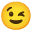# Cashflow Considerations

In an algorithmic system that handles financial events, it’s useful to consider sign conventions. Willi Brammertz takes the view that cashflow is the most important aspect of accounting and models signs from a cashflow perspective where positive cashflow is signed by a positive sign.The first slide gives signs to flows and to assets and liabilities.

The second slide reflects the traditional world of signs in accounting, where assets and liabilities are positively signed (but positioned on the debit or credit side)

The third slide is the slide following strictly the rules set in the first side. Assets are positive and liabilities negative. It recognizes that P&L is the mirror of equity and therefore the entire P&L gets a negative sign. Revenues which are booked on the credit side get – as a consequence – a positive sign (–) and expenses a negative sign (-+). Equity and profit are residuals. In normal cases (positive profit and positive equity) they get as a consequence a positive sign.

I think the third slide is the most natural expression of signs once we accept inflows as positive.Since we begin with liquidity and track inflows as a positive value, then net cashflows off of the profitLoss statement add to cashAndCashEquivalents balance and the flip side on the balance sheet is the increase of equities through a credit adjustment to equity via retained earnings representation.

WBR: Yes, correct. I think here you take profit and equity as a residual.

The math you use for constructing contracts looked interesting. Are you aware of courseware that might allow someone to understand the math & symbols?

WBR: I hope the explanation above helps better. I don’t know of a special book on signs except mine (which however does not discuss signs in depth).

In context of constructing contracts do you have some examples of -+=-  & –++ with respect to liabilities? I’m believe that a cashflow into an entity to pay off a loan reduces liability balance i.e. reduces negative polarity.

WBR: First it is important to recognize, that all financial contracts have in and outflows (Operational contracts have only an in- or an out-flow). For this reason, I say, that the sign of financial contracts are determined by the future flows. A liability has first an inflow followed by outflows (Saving and current accounts have continuous in- and out-flows but the argument still holds). With assets it is the other way round. Example of the liability, say a simple bullet bond (PAM in ACTUS language) of notional 1000: Before value date or the first flow, the future expected principal flows are +1000 and -1000 and they sum up to zero. The value is zero. As soon as the inflow of +1000 at value date comes, we have only future outflow in front of us. The value is -1000. At maturity date we have an outflow of -1000 and no future flows anymore and the value is zero. If we include interest payments (in normal time where rates are positivewe have a sequence of outflows (=expenses) at each interest payment point. Operational contracts have after the first flow no contract related in- or out-flows. This means the future cash-flow is zero. For this reason, their notional value is zero and the value is represented purely by what we call PD.